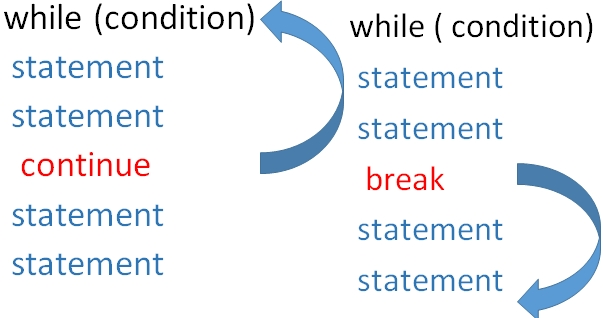# while loop in C programmingUsing while loop we will check the condition first and then execute the statement. Here the C statements can be executed only if condition is True or satisfied.

Usually the condition changes during the execution of the statement so next time while checking the condition we may get a False output. What will happen if the condition is never fails ( always satisfied ) ? We will read it here . Let us start with a basic program to print number 1 to 5.
``````#include<stdio.h>
int main()
{
int i=1;
while (i <=5){
printf("%d  \n",i); // Output
i=i+1; // increment of i by 1
}
return 0;
}``````
The above code will give this output.
``````1
2
3
4
5``````

## Indefinite loop

If we don’t increment the value of I inside the while loop, then the condition will be true in all cases and the loop will never end. This will continuously execute the code inside the loop without any end.
Avoid such loops as they never stops.
``````#include<stdio.h>
int main()
{
int i=1;
while (i <=5){
printf("%d  \n",i); // Output
}
return 0;
}``````
Sometime even the increment conditions became in such a way that the condition never became false and it became indefinite loop.
``````#include<stdio.h>
int main()
{
int i=1;
while (i <=5){
printf("%d  \n",i); // Output
i=i-1; // decrement of i by 1
}
return 0;
}``````

## Break statement

We can terminate the execution of loop in between and came out of the loop by using break statement.
Here we have used one if condition checking and once the value of i became 4 then break; statement gets executed and the loop is terminated.
``````#include <stdio.h>
int main(void){
int i=1;

while(i <= 5){
printf("\n I value before increment  : %d  ", i);
i=i+1; // increment of i by 1

if(i==4){
break;
}
printf("\n I value after increment  : %d  ", i);
}

return 0;
}``````
Output is here
`````` I value before increment  : 1
I value after increment  : 2
I value before increment  : 2
I value after increment  : 3
I value before increment  : 3``````
Note that i value 4 and i value 5 is not printed as break; statement stopped the execution of the loop.

## continue statement

Using continue; statement we can skip the execution of code blok in rest of the loop, however the loop will continue the execute from starting.

## Difference between break and continue

After encountering break; statement the execution will come out of the loop. In case of continue; statement the execution for rest of the loop is skipped and the execution will start from starting of the loop ( checking of condition ).We will use the same code as above and in place of break; we used continue; here
``````while(i <= 5){
printf("\n I value before increment  : %d  ", i);
i=i+1; // increment of i by 1

if(i==4){
continue;
}
printf("\n I value after increment  : %d  ", i);
}``````
Output
`````` I value before increment  : 1
I value after increment  : 2
I value before increment  : 2
I value after increment  : 3
I value before increment  : 3
I value before increment  : 4
I value after increment  : 5
I value before increment  : 5
I value after increment  : 6``````
Note that one step is skiped. The step to show `I value after increment : 4` is not displayed ( skipped ) but execution continued from staring of the loop again and displayed next value.

## incrementing or decrementing steps

We will increment the variable by more steps.
``````i++; // increment i value by 1
i--; // decrement i value by 1
i += 5; // increment i value by 5
i -= 5; // Decrement i value by 5 ``````

## Example with decrement of value by 10

``````#include<stdio.h>
int main()
{
int i=50;
while (i >= 0){
printf("%d  \n",i); // Output
i-=10; // increment of i by 1
}
return 0;
}``````
Output is here
``````50
40
30
20
10
0``````

## Reversing a string without using strlen()

strlen()
``````#include<stdio.h>
#include<string.h>
int main()
{
char str="plus2net";
int str_length;
str_length=strlen(str); // length of the string
while(str_length>=0){
printf("%c",str[str_length]);
str_length--;
}
return 0;
}``````

## Use of While loop in C

Sum of user entered numbers till 0 is entered by user.

## Subscribe

* indicates required
Subscribe to plus2netplus2net.com

 21-01-2020 Write a program that finds the sum of even numbers between zero and twenty use while loop